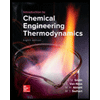Question

The oxidative coupling of methane to form ethylene (2 CH4 + O2 -> C2H4 + 2 H2O) is known as the “holy grail of catalysis.” For decades, researchers around the world have worked to develop a catalyst that can perform the reaction at an industrial level, but no one has succeeded in that task. You believe that you have found the perfect lanthanide catalyst and you are preparing your Nobel Prize acceptance speech, but first, you have to determine various values regarding your inlet stream. Experimentally, you have found the best conversion and selectivity from an inlet mixture of 37.5% methane, 12.5% O2, and 50% argon, by volume, and at 650°C and 4 atm. If your inlet mixture follows the ideal gas, determine:

a. Each component’s partial pressure.

b. Each component’s mass fraction and the inlet mixture’s average molecular weight.

c. The inlet mixture’s density (in kg/m3).

d. Is this mixture well-modeled by the ideal gas law? Why or why not?

e. You want to find the density using the truncated virial equation of state (use Equation 5.3-3 in the textbook). Without solving, write the equation to solve for the inlet mixture’s density using the virial equation of state, in terms of R, T, P, B, and molecular weight.

Equation 5.3-3: (PV(hat)/RT)=1+(BP/RT)

Expert Solution

### Want to see the full answer?

Check out a sample Q&A hereStudents who’ve seen this question also like:Introduction to Chemical Engineering Thermodynamics
8th Edition
ISBN: 9781259696527
Author: J.M. Smith Termodinamica en ingenieria quimica, Hendrick C Van Ness, Michael Abbott, Mark Swihart
Publisher: McGraw-Hill Education